Скачать презентацию A Modular Checker for Multithreaded Programs Cormac Flanagan

• Количество слайдов: 23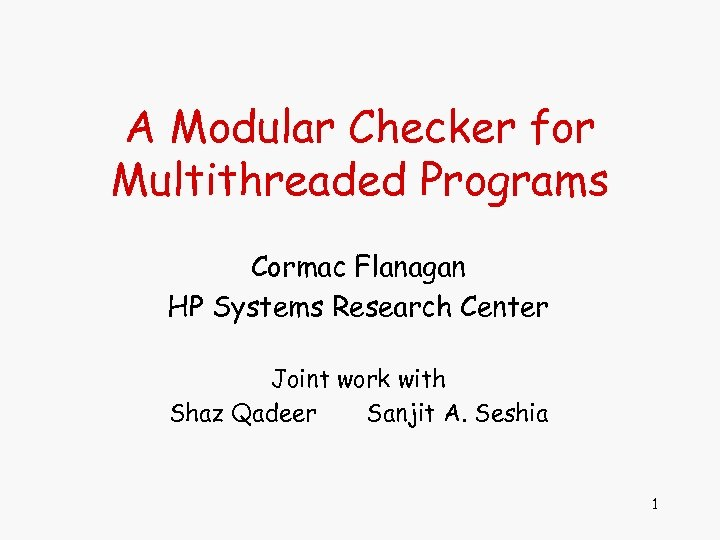A Modular Checker for Multithreaded Programs Cormac Flanagan HP Systems Research Center Joint work with Shaz Qadeer Sanjit A. Seshia 1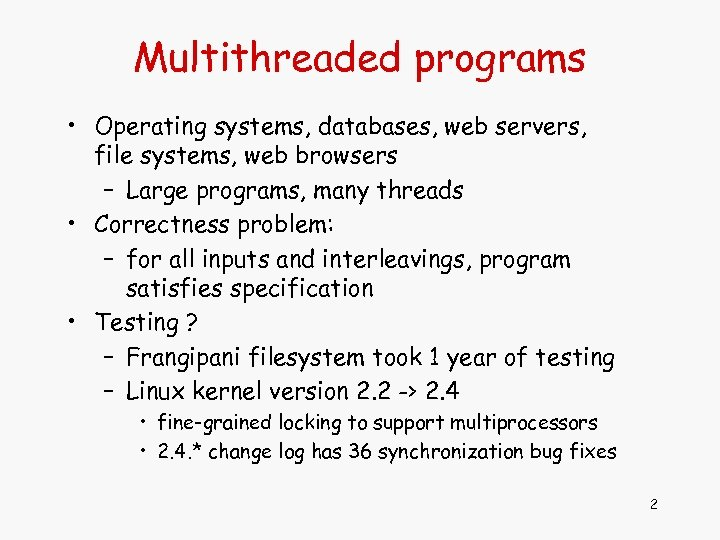Multithreaded programs • Operating systems, databases, web servers, file systems, web browsers – Large programs, many threads • Correctness problem: – for all inputs and interleavings, program satisfies specification • Testing ? – Frangipani filesystem took 1 year of testing – Linux kernel version 2. 2 -> 2. 4 • fine-grained locking to support multiprocessors • 2. 4. * change log has 36 synchronization bug fixes 2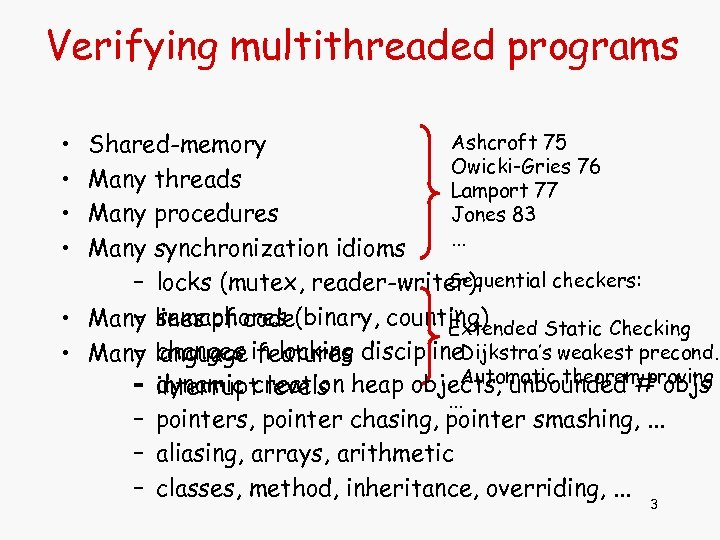Verifying multithreaded programs • • Ashcroft 75 Shared-memory Owicki-Gries 76 Many threads Lamport 77 Jones 83 Many procedures. . . Many synchronization idioms Sequential checkers: – locks (mutex, reader-writer). . . – semaphores • Many lines of code(binary, counting) Extended Static Checking – changes features • Many language in locking discipline. Dijkstra’s weakest precond. Automatic theorem proving dynamic creation – interrupt levels heap objects, unbounded # objs. . . – pointers, pointer chasing, pointer smashing, . . . – aliasing, arrays, arithmetic – classes, method, inheritance, overriding, . . . 3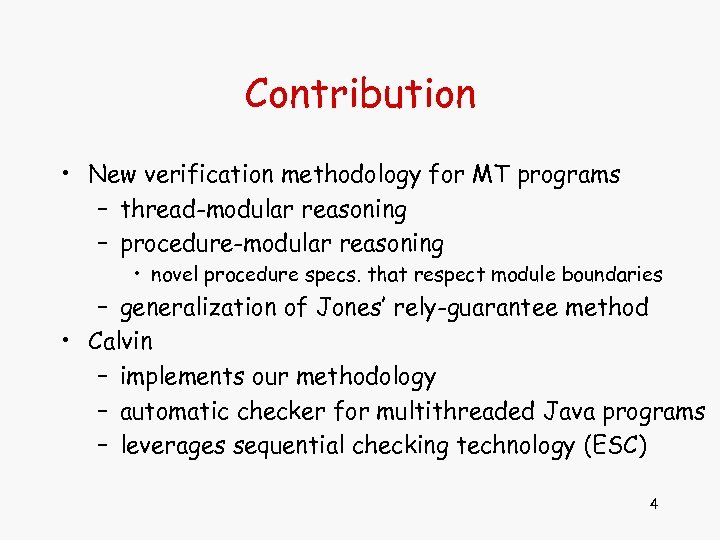Contribution • New verification methodology for MT programs – thread-modular reasoning – procedure-modular reasoning • novel procedure specs. that respect module boundaries – generalization of Jones’ rely-guarantee method • Calvin – implements our methodology – automatic checker for multithreaded Java programs – leverages sequential checking technology (ESC) 4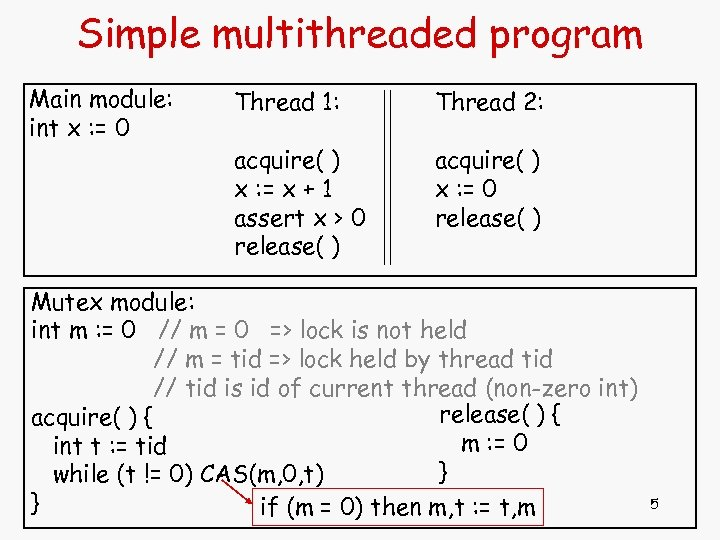Simple multithreaded program Main module: int x : = 0 Thread 1: Thread 2: acquire( ) x : = x + 1 assert x > 0 release( ) acquire( ) x : = 0 release( ) Mutex module: int m : = 0 // m = 0 => lock is not held // m = tid => lock held by thread tid // tid is id of current thread (non-zero int) release( ) { acquire( ) { m : = 0 int t : = tid } while (t != 0) CAS(m, 0, t) } if (m = 0) then m, t : = t, m 5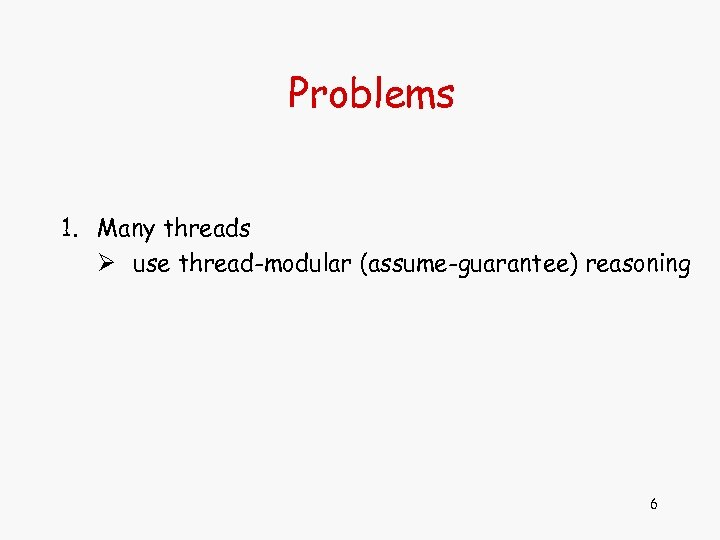Problems 1. Many threads Ø use thread-modular (assume-guarantee) reasoning 6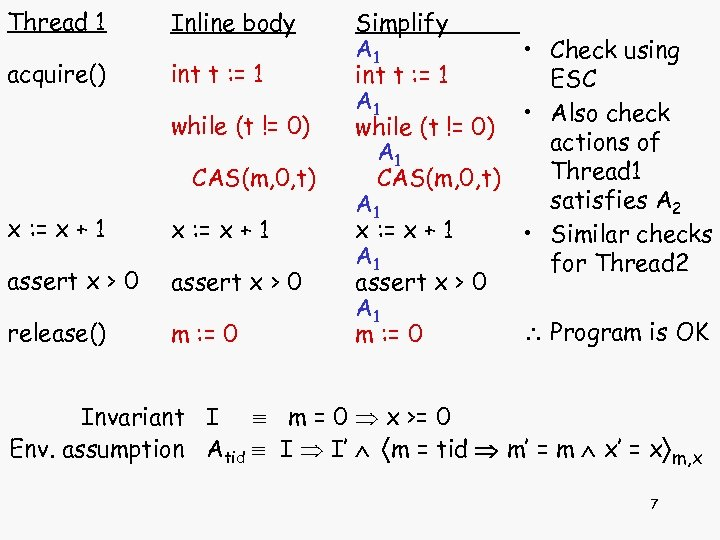Thread 1 Inline body acquire() int t : = 1 while (t != 0) CAS(m, 0, t) x : = x + 1 assert x > 0 release() m : = 0 Other threads Env assumption Simplify A 1* • Check using 1* int t : = 1 ESC A 1* 1* • Also check while (t != 0) actions of A 1 * 1 Thread 1 CAS(m, 0, t) satisfies A 2 A 1* 1* x : = x + 1 • Similar checks A 1* 1* for Thread 2 assert x > 0 A 1* 1* Program is OK m : = 0 Invariant I m = 0 x >= 0 Env. assumption Atid I I’ m = tid m’ = m x’ = x m, x 7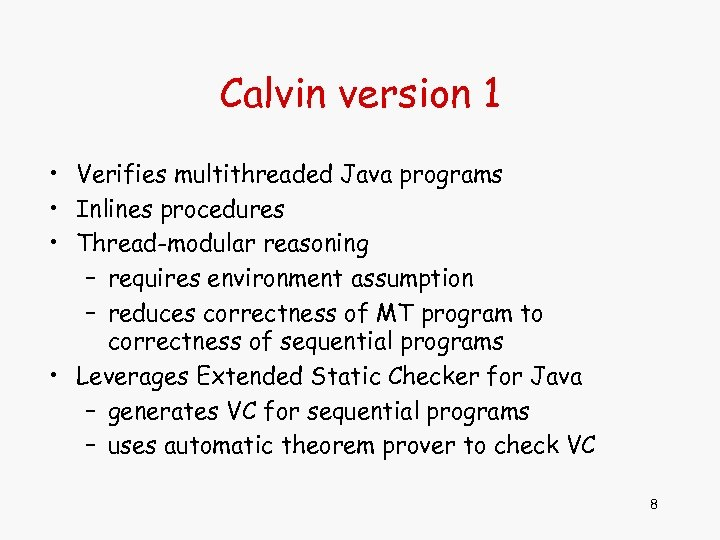Calvin version 1 • Verifies multithreaded Java programs • Inlines procedures • Thread-modular reasoning – requires environment assumption – reduces correctness of MT program to correctness of sequential programs • Leverages Extended Static Checker for Java – generates VC for sequential programs – uses automatic theorem prover to check VC 8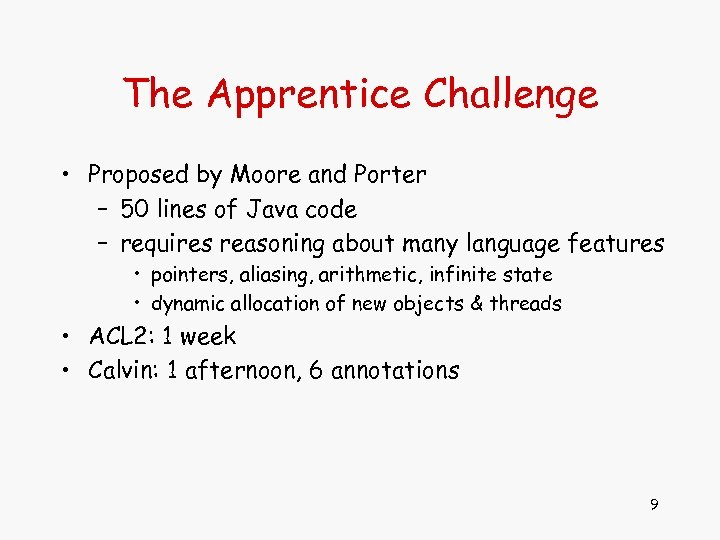The Apprentice Challenge • Proposed by Moore and Porter – 50 lines of Java code – requires reasoning about many language features • pointers, aliasing, arithmetic, infinite state • dynamic allocation of new objects & threads • ACL 2: 1 week • Calvin: 1 afternoon, 6 annotations 9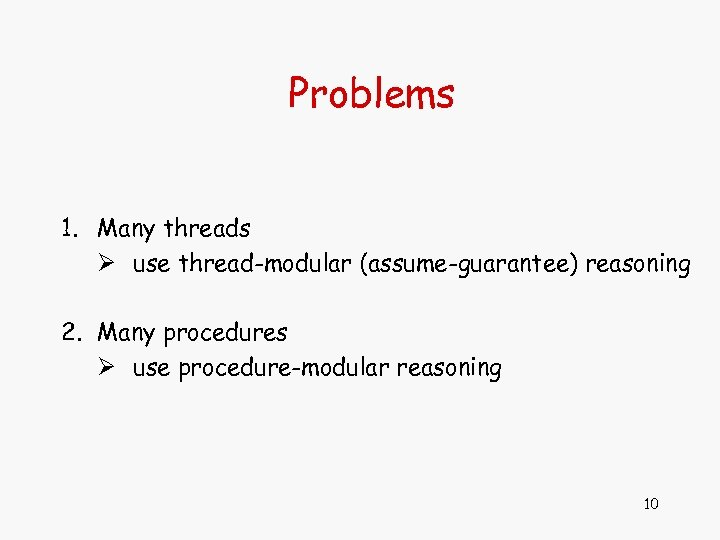Problems 1. Many threads Ø use thread-modular (assume-guarantee) reasoning 2. Many procedures Ø use procedure-modular reasoning 10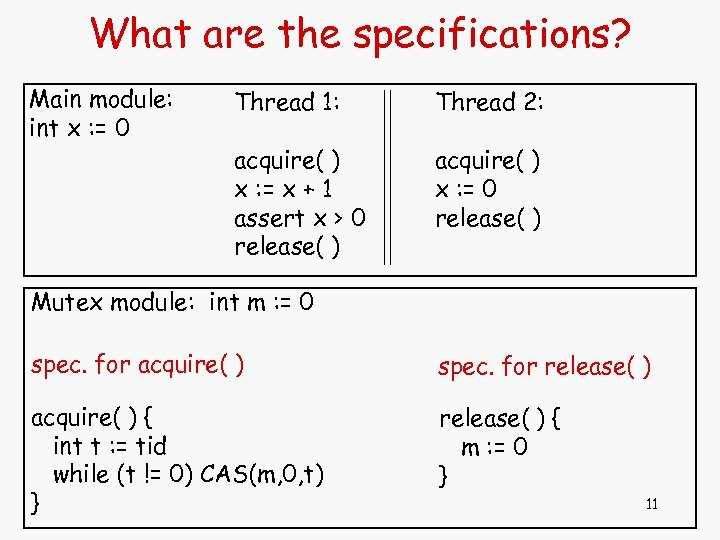What are the specifications? Main module: int x : = 0 Thread 1: Thread 2: acquire( ) x : = x + 1 assert x > 0 release( ) acquire( ) x : = 0 release( ) Mutex module: int m : = 0 spec. for acquire( ) spec. for release( ) acquire( ) { int t : = tid while (t != 0) CAS(m, 0, t) } release( ) { m : = 0 } 11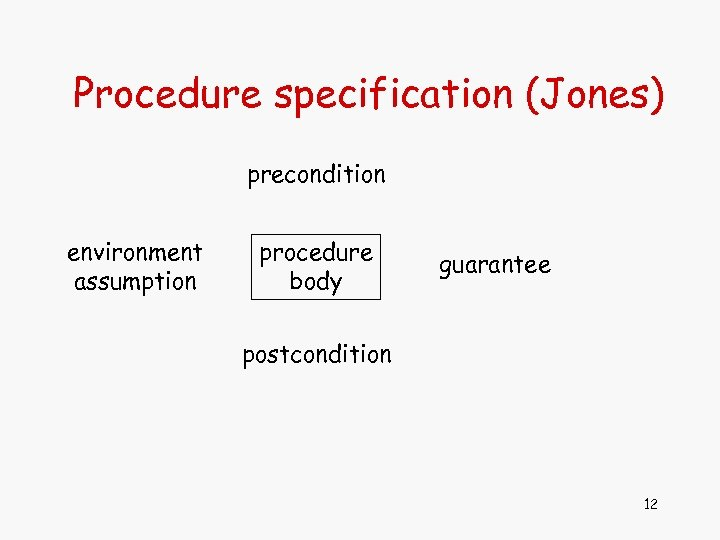Procedure specification (Jones) precondition environment assumption procedure body guarantee postcondition 12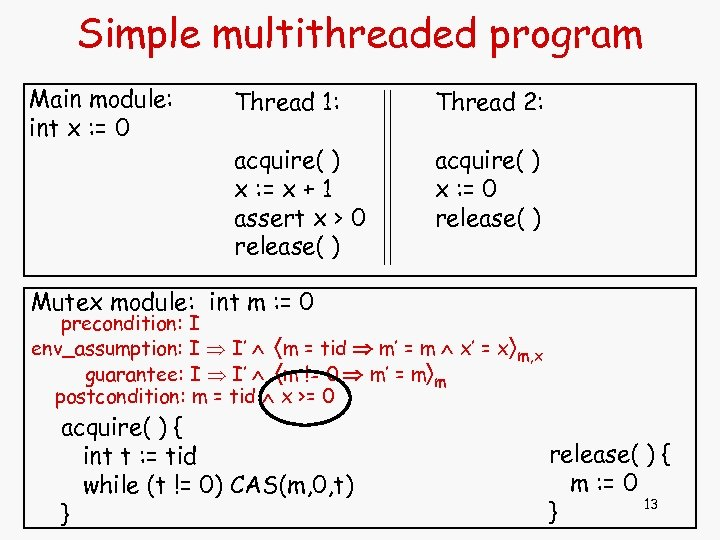Simple multithreaded program Main module: int x : = 0 Thread 1: Thread 2: acquire( ) x : = x + 1 assert x > 0 release( ) acquire( ) x : = 0 release( ) Mutex module: int m : = 0 precondition: I env_assumption: I I’ m = tid m’ = m x’ = x m, x guarantee: I I’ m != 0 m’ = m m postcondition: m = tid x >= 0 acquire( ) { int t : = tid while (t != 0) CAS(m, 0, t) } release( ) { m : = 0 13 }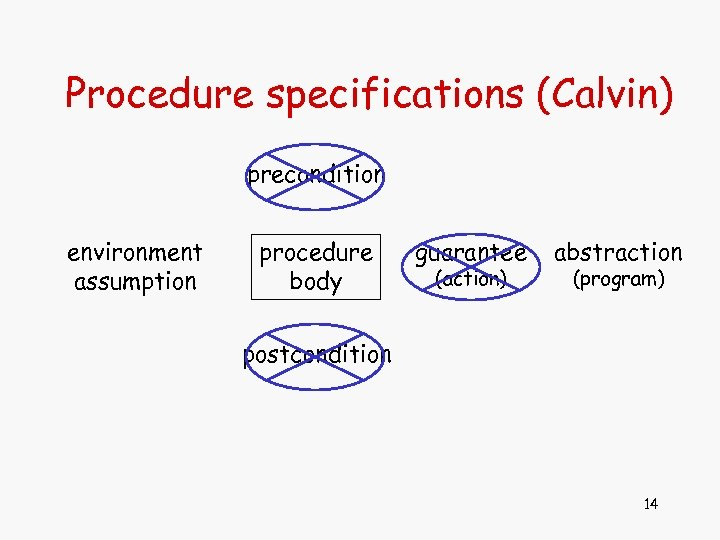Procedure specifications (Calvin) precondition environment assumption procedure body guarantee (action) abstraction (program) postcondition 14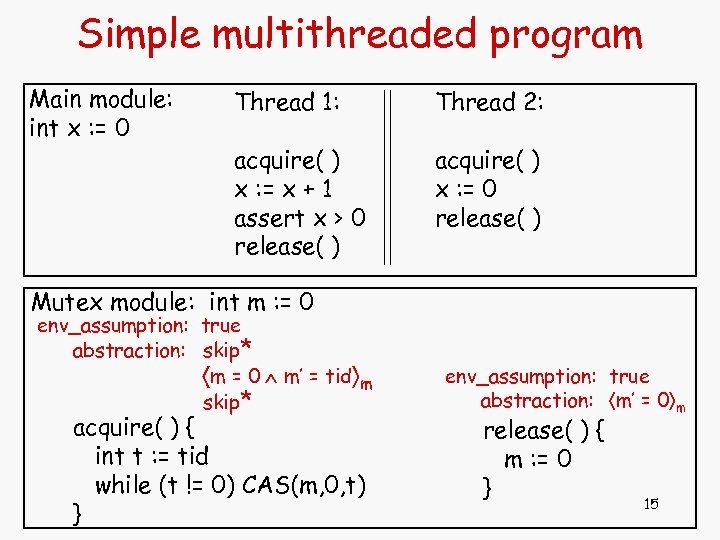Simple multithreaded program Main module: int x : = 0 Thread 1: Thread 2: acquire( ) x : = x + 1 assert x > 0 release( ) acquire( ) x : = 0 release( ) Mutex module: int m : = 0 env_assumption: true abstraction: skip* m = 0 m’ = tid m skip* acquire( ) { int t : = tid while (t != 0) CAS(m, 0, t) } env_assumption: true abstraction: m’ = 0 m release( ) { m : = 0 } 15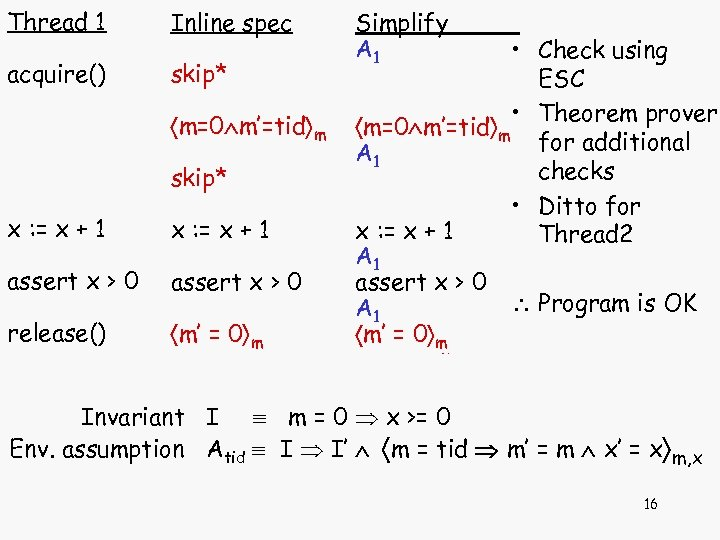Thread 1 Inline spec acquire() skip* m=0 m’=tid m skip* x : = x + 1 assert x > 0 release() m’ = 0 m Env assumption Other threads Simplify A 1* • Check using 1* skip* ESC A 1* * • Theorem prover m=0 m’=tid m m for additional A 1 * * 1 checks skip* • Ditto for A 1* * x : = x + 1 Thread 2 x : = x + 1 A 1* 1* assert x > 0 Program is OK A 1* 1* m’ = 0 m m Invariant I m = 0 x >= 0 Env. assumption Atid I I’ m = tid m’ = m x’ = x m, x 16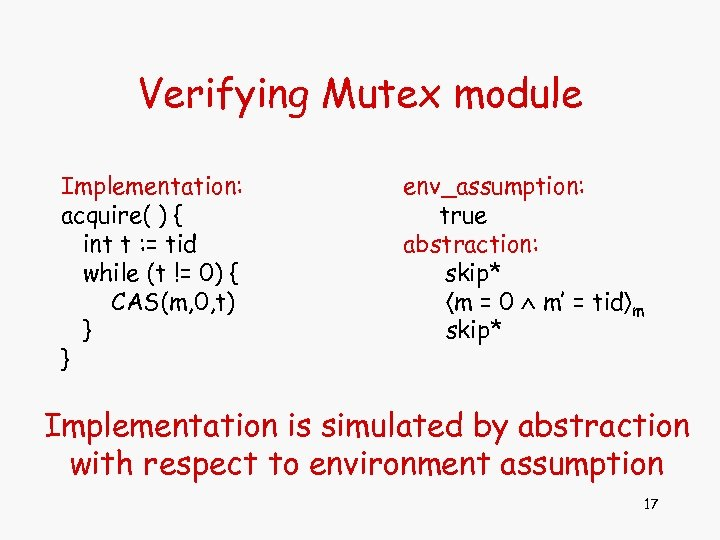Verifying Mutex module Implementation: acquire( ) { int t : = tid while (t != 0) { CAS(m, 0, t) } } env_assumption: true abstraction: skip* m = 0 m’ = tid m skip* Implementation is simulated by abstraction with respect to environment assumption 17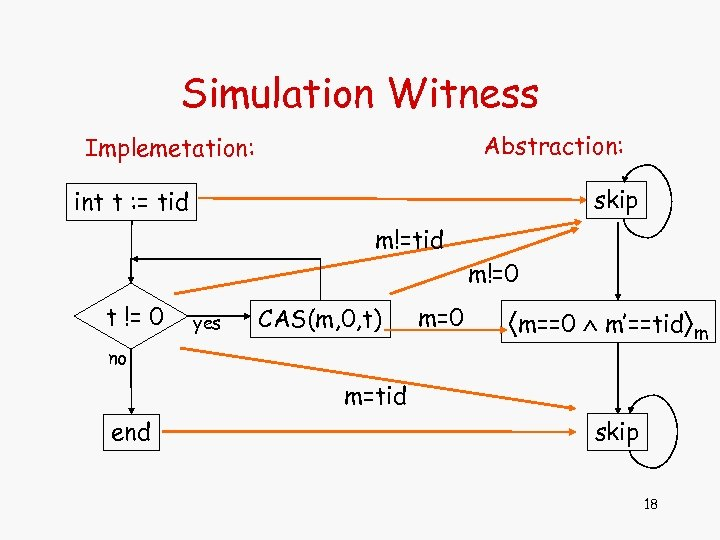Simulation Witness Abstraction: Implemetation: skip int t : = tid m!=0 t != 0 yes CAS(m, 0, t) m=0 m==0 m’==tid m no m=tid end skip 18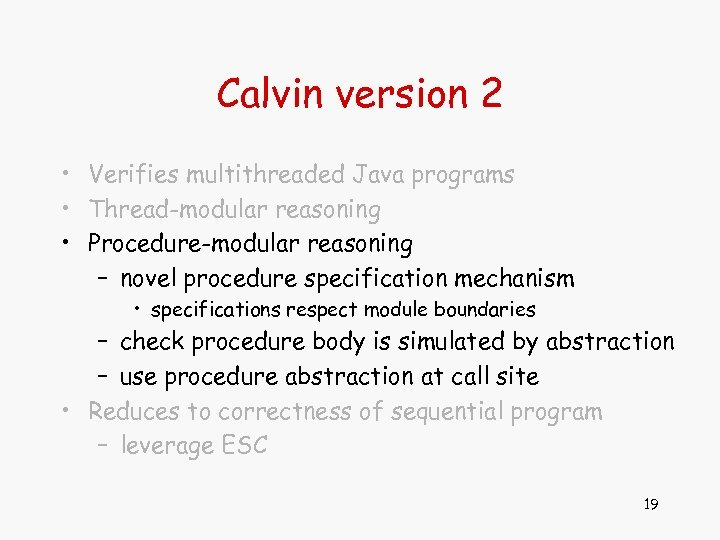Calvin version 2 • Verifies multithreaded Java programs • Thread-modular reasoning • Procedure-modular reasoning – novel procedure specification mechanism • specifications respect module boundaries – check procedure body is simulated by abstraction – use procedure abstraction at call site • Reduces to correctness of sequential program – leverage ESC 19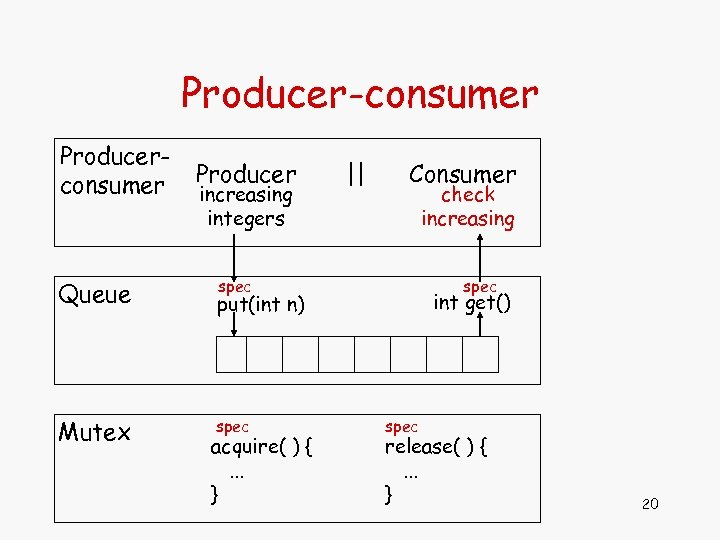Producer-consumer Producerconsumer Queue Mutex Producer increasing integers || Consumer check increasing spec int get() put(int n) spec acquire( ) {. . . } spec release( ) {. . . } 20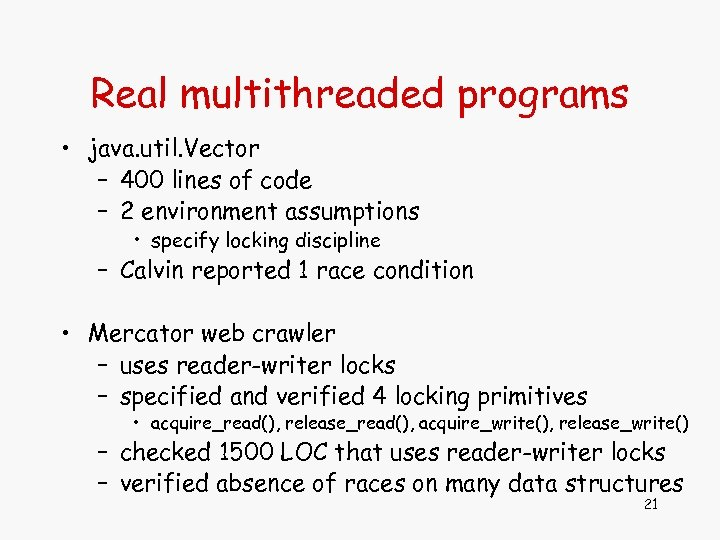Real multithreaded programs • java. util. Vector – 400 lines of code – 2 environment assumptions • specify locking discipline – Calvin reported 1 race condition • Mercator web crawler – uses reader-writer locks – specified and verified 4 locking primitives • acquire_read(), release_read(), acquire_write(), release_write() – checked 1500 LOC that uses reader-writer locks – verified absence of races on many data structures 21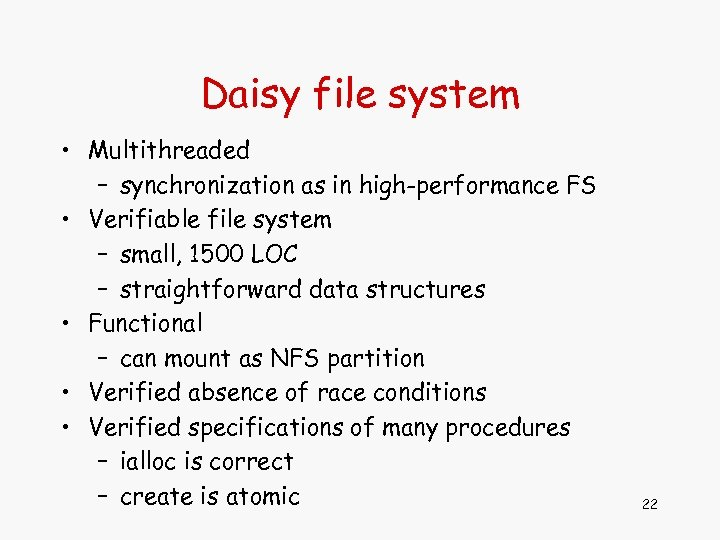Daisy file system • Multithreaded – synchronization as in high-performance FS • Verifiable file system – small, 1500 LOC – straightforward data structures • Functional – can mount as NFS partition • Verified absence of race conditions • Verified specifications of many procedures – ialloc is correct – create is atomic 22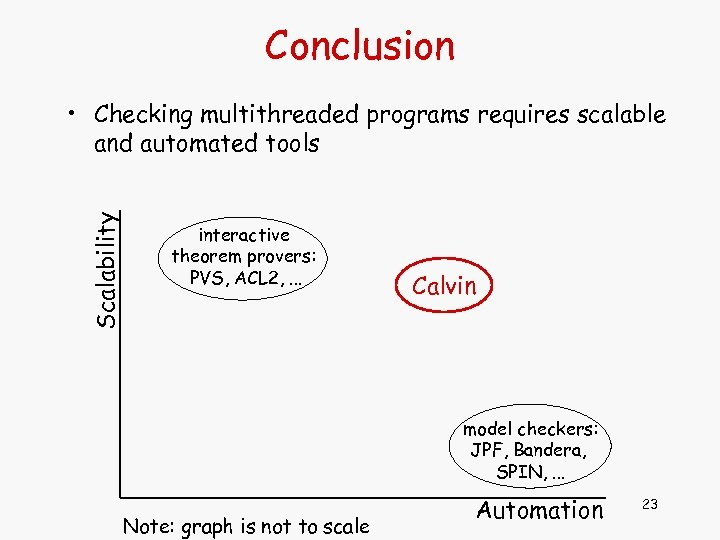Conclusion Scalability • Checking multithreaded programs requires scalable and automated tools interactive theorem provers: PVS, ACL 2, . . . Calvin model checkers: JPF, Bandera, SPIN, . . . Note: graph is not to scale Automation 23